# Events

Two simba ways to share:

## What methods exist for calculating the capacity of a shear wall line when there are multiple segments of unequal length?

The American Wood Council’s (AWC) 2015 Special Design Provisions for Wind and Seismic (SDPWS) Section 4.3.3.3.4 provides two options for determining shear distribution to wood-sheathed shear walls in the same line of lateral force resistance when using the segmented shear wall approach. One method is by equal deflection and the other is per distribution of shear in proportion to shear wall length.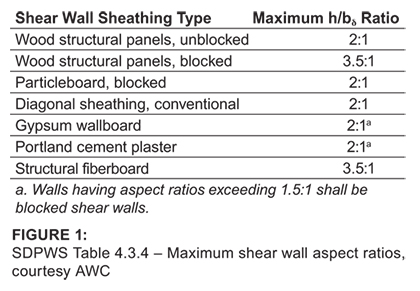One of the first steps in calculating the capacity for a line of segmented shear walls is to determine which segments in the wall line qualify for use.  Table 4.3.4 (Figure 1) gives maximum shear wall aspect ratios for various types of sheathed walls.  For blocked wood structural panels, the maximum aspect ratio is 3.5:1.  For segments with an aspect ratio between 2:1 and 3.5:1, the nominal shear capacity will need to be multiplied by the Aspect Ratio Factor, which is 1.25 – 0.125h/bs for wood structural panels (SDPWS 4.3.4.2).  Segments with an aspect ratio greater than 3.5:1 cannot be used to resist lateral forces, as shown in Figure 2.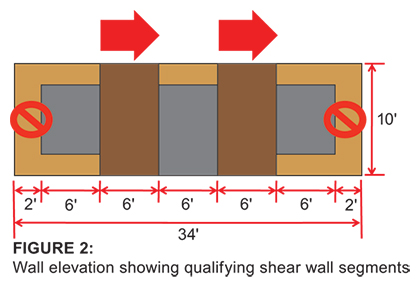Where shear wall segments are the same length, simply dividing the total force by the number of segments will provide the force for each one.  Unit shear is also easily determined by dividing the total shear force by the sum of resisting segment lengths.  From there, the appropriate sheathing, blocking and nailing pattern for the wall can be specified per SDPWS Section 4.3 provisions.  For a shear wall line with segments of unequal widths (Figure 3), calculation of the forces to each segment is more involved.

The Commentary to Chapter 4 of the SDPWS includes two calculation methods for this scenario—the equal deflection method, shown in Example C4.3.3.4.1-1, and the simplified method, illustrated in Example C4.3.3.4.1-2.

## EQUAL DEFLECTION METHODSDPWS 4.3.3.4.1 states that shear distribution to individual shear walls in a shear wall line shall provide the same calculated deflection, δsw, in each shear wall.  The equal deflection method has the basic assumption that the distribution of forces to each wall segment will be based on the relative stiffness of each segment.  As the shear wall segments are connected, when the diaphragm transfers shear to the wall line, the segments are assumed to deflect equally.

Equal Deflection Method

• δSW1 = δSW2

The total design capacity of the shear wall line will be the sum of the resisting forces in each shear wall at the calculated deflection.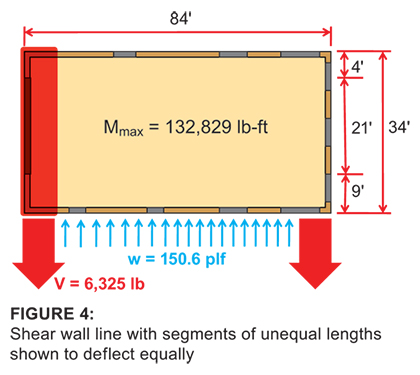As an example, let’s consider a building that is 84×34 feet in plan, single story, with 10-foot-tall exterior walls.  The first check is to determine the shear wall segment aspect ratios to make sure they fall within the limits detailed in SDPWS Table 4.3.4.

Shear Wall aspect ratios:

SW1 = 10ˈ/4ˈ = 2.5  <  3.5   OK
SW2 = 10ˈ/9ˈ = 1.1  <  3.5   OK

Checking the ratios, we find that both shear wall segments fall within allowable limits.  Also note that the aspect ratio of SW1 is greater than SW2, so its capacity will need to be adjusted per SDPWS 4.3.4.2.  Our objective is to calculate the maximum wall strength (capacity) based on the maximum nominal shears for the wall construction, which can then be compared to actual demand.

For shear wall design in this example, we assume 15/32-inch OSB, Wood Structural Panels – Sheathing, attached with 8d nails @ 3 inches on center to 2×6 Spruce Pine Fir (SPF) framing spaced 16 inches on center with two 2×6 posts at the end of each wall segment.  Forces from wind loading are shown in Figure 4. The wall line in question is the left wall on the plan shown in Figure 4 (elevation view shown in Figure 3).

## SW1

h/bs = 2.5 > 2

Aspect Ratio Factor = 1.25-0.125(h/bs) = 0.938  (SDPWS Section 4.3.4.2)

From SDPWS Table 4.3A:

Nominal unit shear capacity = 1,370 lb/ft

Adjusted ASD capacity = [(1,370 plf)(0.92)/2]*0.938 = 591 lb/ft

0.92 is the adjustment for having SPF framing; see SDPWS Table 4.3A, footnote 3.  Divide by 2 for the ASD allowable unit shear capacity per SDPWS 4.3.3.

## SW2

As our sheathing thickness, blocking and nailing pattern will remain the same for both segments, by observation we recognize that SW2 is the stiffer of the two shear wall segments (longer).  SW2 will have the smaller deflection at its capacity and ultimately control the deflection of both shear walls.

h/bs = 1.11 < 2  OK

Nominal unit shear capacity = 1,370 lb/ft  (SDPWS Table 4.3A)

Adjusted ASD capacity = (1,370 plf)(0.92)/2 = 630 lb/ft

We now calculate the deflection of SW2 at its ASD unit shear capacity:v = 630 lb/ft
E = 1,400,000 psi   (NDS* Supplement Table 4A)
A = 2(1.5″x5.5″) = 16.5 in2  (2-2×6 stud end post)
b = 9ˈ
h = 10ˈ
Ga = 14 k/in   (SDPWS Table 4.3A)
Δa = vertical elongation of wall anchorage = 0.091″ at 6,560 lbs (from hold down manufacturer)
SW2 anchorage force = (630 lb/ft)(10’) = 6,300 lb
*Assuming vertical elongation is linear, we can calculate elongation for our load of 6,300 lbs.
Δa = 6,300 * 0.091″ / 6,560 lb = 0.087″

δSW2 = 0.571

Determine the unit shear in SW1 that produces the same deflection as SW2:

k = stiffness of the anchorage = F / d (deflection / elongation)

k = 6,560 lbs / 0.091″ = 72,087 lb/in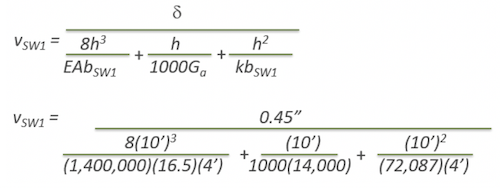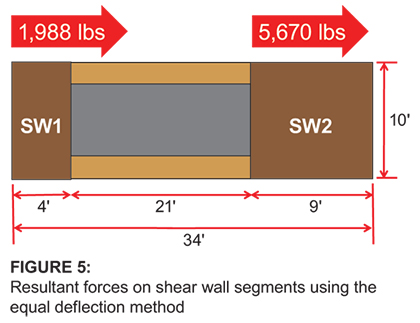vSW1 =  497 lb/ft  <  591 lb/ft

Shear wall line capacity:

V = (497 lb/ft)*4ˈ + (630 lb/ft)*9ˈ
V = 1,988 lbs + 5,670 lbs
V = 7,658 lb  >  6,325 lb Actual  OK

SIMPLIFIED METHOD

For wood structural panels, distribution of shear in proportion to shear strength (by wall length) of each shear wall is permitted provided shear walls with aspect ratios greater than 2:1 have strength adjusted by the 2bs/h factor (per 2015 SDPWS 4.3.3.4.1, Exception 1)Using this method:

## SW1

SW1 Aspect Ratio = 2.5 > 2  Nominal shear capacity will need to be adjusted by 2bs /h per SDPWS 4.3.3.4.1 Exception 1.

h/bs = 2.5 > 2
2bs /h = 2*(4ˈ/10ˈ) = 0.8
Nominal unit shear capacity = 1,370 lb/ft  (SDPWS Table 4.3A)
Adjusted ASD capacity = [(1,370 plf)(0.92)/2]*0.8 = 504 lb/ft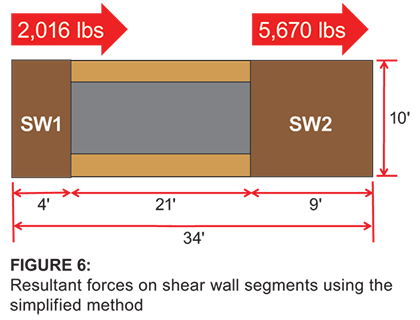## SW2

h/bs = 1.1 < 2
Nominal unit shear capacity = 1,370 lb/ft  (SDPWS Table 4.3A)
Adjusted ASD capacity = (1,370 plf)(0.92)/2 = 630 lb/ft

Shear wall line capacity:

V = (504 lb/ft)*4ˈ + (630 lb/ft)*9ˈ
V = 2,016 lbs + 5,670 lbs
V = 7,686 lb  >  6,325 lb Actual  OK

Comparing the two methods we see a slight increase in the resistance provided by the simplified method.Beyond the segmented shear wall approach, SDPWS Section 4.3.5 describes two other methods for evaluating shear wall lateral resistance—the Perforated Shear Wall (PSW) method and Force Transfer Around Openings (FTAO) method.  For further reading on these two methods, see the resources listed below.